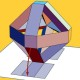#### [SOLVED] Concentration of measure for arbitrary convex bodies?

There are various "concentration-of-measure" theorems, the best known that due to Lévy, which is this (informally): the volume of a sphere $S^d$ in $d$ dimensions is largely concentrated around an $\epsilon$-tubular neighborhood of an equitorial hyperplane $H$. Here the tubular neighborhood is the set of points within distance $\epsilon$ of $H$. I believe there is an analogous theorem for centro-symmetric convex bodies (although I have no reference for this). My question is:

Is there a concentration-of-measure theorem for arbitrary convex bodies $K$, something along these lines: There exists a hyperplane $H$ such that most of the volume of $K$ is concentrated in a tubular neighborhood of $H$?

Or are there convex bodies so "skewed" that they resist any such section?

Likely this is known, in which case a reference would suffice. Thanks!#### @Mark Meckes 2011-06-14 14:29:28

There are many results, and an active research industry, along these lines. In general the Euclidean ball is the best-behaved convex body in this respect, and just how similar an arbitrary convex body is depends on how you try to make your question more precise. Here's one very general result, a special case of what is sometimes called Borell's lemma:

Let $K \subset \mathbb{R}^n$ be a convex body with volume 1 and let $A \subset \mathbb{R}^n$ be a convex symmetric set such that $vol(K\cap A) \ge 3/4$. Then for $r > 1$, $$vol(K \setminus r A) \le \frac{3}{4} 3^{-(r+1)/2}.$$

Thus in particular the volume of $K$ far from a hyperplane decays exponentially.

Much sharper results exist. I mentioned a couple recent references in a comment on Gil's answer. For much more, I suggest you start by perusing the papers of Grigoris Paouris.#### @Gil Kalai 2011-06-13 17:14:56

The paper "Isoperometric problems for convex bodies and a localization lemme" by Kannan Lovasz and Simonovitz (Disc Comp Geom 13(1995) 541-559) seems relevant. They consider the minimum volume of a (n-1) dimensional surface needed to seperate a convex body to sets of large volume each. If the volume is large then so is the volume of a tobular neighborhood.#### @Joseph O'Rourke 2011-06-13 18:54:35

@Gil: Very interesting! They have a nice conjecture relating the volume of the minimal separating surface to the largest eigenvalue of an inertia matrix. This seems to be a way to generalize from sphere to centro-symmetric to arbitrary convex body. Thanks!#### @Joseph O'Rourke 2011-06-13 22:14:45#### @Mark Meckes 2011-06-14 14:16:48

@Joseph: This conjecture is now very famous and frequently referred to as the KLS conjecture. For the current state of affairs, you can look at recent work of Klartag, e.g. math.tau.ac.il/~klartagb/papers/thin_slice6.pdf and Guedon and E. Milman: arxiv.org/abs/1011.0943

### [SOLVED] Convex misfit of finite-dimensional convex bodies

• 2018-06-07 05:12:08
• Wlod AA
• 120 View
• 2 Score